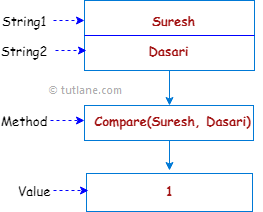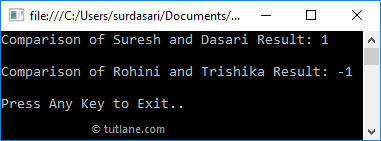# Visual Basic String Compare Method

In visual basic, the string Compare method is useful to compare two specified strings and return an integer value that indicates their relative position in the sort order.

Here, the return integer value indicates the lexical relationship between the two specified strings. The following table lists the values which will be returned by the Compare method after comparing the two specified strings.

ConditionValue
strA == strB 0
strA > strB 1
strA < strB -1

Following is the pictorial representation of comparing two strings using the Compare() method in a visual basic programming language.If you observe the above diagram, we are comparing two strings ”Suresh” and “Dasari” by using the Compare method and return an integer value “1”.

## Visual Basic String Compare Method Syntax

Following is the syntax of defining a Compare method to compare two specified strings in a visual basic programming language.

Public Function Compare(ByVal string1 As String, ByVal string2 As String) As Integer

If you observe the syntax, the Compare method will compare the given two strings and return an integer value.

## Visual Basic String Compare Method Example

Following is the example of using the Compare() method to compare a given two strings and return an integer value in a visual basic programming language.

Module Module1

Sub Main()

Dim str1 As String = "Suresh"

Dim str2 As String = "Dasari"

Console.WriteLine("Comparison of {0} and {1} Result: {2}", str1, str2, String.Compare(str1, str2))

Dim str3 As String = "Rohini"

Dim str4 As String = "Trishika"

Console.WriteLine("Comparison of {0} and {1} Result: {2}", str3, str4, String.Compare(str3, str4))

Console.WriteLine("Press Enter Key to Exit..")

End Sub

End Module

If you observe the above example, we used a Compare() method to compare two given strings and return an integer value.

When we execute the above visual basic program, we will get the result as shown below.This is how we can use Compare() method to compare two given strings and return an integer value which indicates a lexical relationship between two strings in a visual basic programming language.